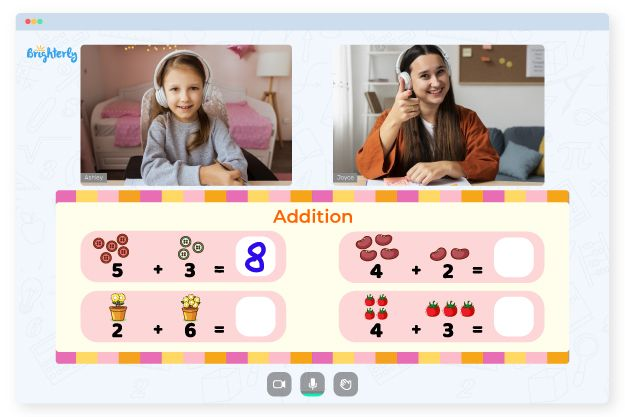# Adding, Subtracting, Multiplying And Dividing Fractions Worksheets

If your child uses an add subtract multiply divide fractions worksheet with exercises, they will enhance their skills of working with basic mathematics operations. It’s significant for kids to work with worksheets of various difficulty. With consistency and continuous practice, worksheets will make your kid more interested in math and better at problem-solving.

This fraction worksheet combines exercises on four operations and helps your kids understand them as separate fundamental concepts. These operations are intertwined, and mastering them will require your child to gain essential skills like mass deletion, sorting, manipulating, and using equivalence. However, they have nothing to worry about as this worksheet is designed to make the process of gaining those skills easy and quick.

Math for Kids

Is Your Child Struggling With Math?
1:1 Online Math Tutoring## Benefits of Add Subtract Multiply and Divide Fractions Worksheet

These worksheets are the best tools for children who want to improve their math grades. The worksheets contain exercises that help kids perform like they are in an examination environment. For each of the operations, there are creative, logical, and exciting activities that help improve a kid’ number senses.### Adding, Subtracting, Multiplying And Dividing Fractions Worksheets PDF

Adding, Subtracting, Multiplying And Dividing Fractions Worksheet### Adding, Subtracting, Multiplying And Dividing Fractions Worksheets PDF

Add, Subtract, Multiply And Divide Fractions Worksheet### Adding, Subtracting, Multiplying And Dividing Fractions Worksheets PDF

Add, Subtract, Multiply, Divide Fractions Worksheet### Adding, Subtracting, Multiplying And Dividing Fractions Worksheets PDF

Adding, Subtracting, Multiplying And Dividing Fractions Worksheet Pdf

Adding, subtracting, multiplying, and dividing fractions worksheets give kids the ability to work on different math problems with much ease. Thus, they can understand basic arithmetic concepts, from simple operations to advanced ones. The worksheets are easy to use, understand, and work upon, as well as self-explanatory, which makes learning easy.

### More Worksheets• Try lessons with an online tutor.

Is your child having difficulties with understanding addition? An online tutor could provide the necessary support.